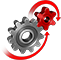# WolframSystemModeler

## Pong Game

This example will demonstrate how you can use Model Plug to create your own Pong game, played with external controllers. This is a very playful example, but it highlights how easy the connection to external hardware becomes when using the Model Plug library.

### The Setup

To create a Pong game using System Modeler, you will need a Pong model created in Wolfram System Modeler, the Model Plug library, and some external hardware that will act as paddles in the game.This is the animation of the Pong game simulation. You can see both paddles, the current score (displayed by the bars in the back), and the red ball.Picture of the external hardware. Top left is the button to start the game, top right is the first paddle (slider) for one racket, bottom left is the Arduino board the hardware is connected to, and bottom right is the second paddle (joystick) for the other racket.

### The Model

The System Modeler model consists of connections to external hardware connected to a model of a Pong game. This game, or model, contains mechanical equations of a bouncing ball. When the ball hits either one of the walls or one of the paddles, the ball will bounce, and if a player manages to score, the score will be updated, and the ball will reinitialize to the middle of the board.Above is the diagram view of the Pong model. These components each contain equations that build up the game. By using this component way of structuring your mathematical model, it will be easy to understand how the underlying equations are connected to each other as you create the different parts of the model.

### Playing the Game

Connect External Hardware

Use external hardware to provide input to your running simulation.

This is a video with two people interacting with the Pong simulation in real time, using two sliders to control the two different paddles.

### Expanding the Model

To make the model more interesting and increase the difficulty for the players, you can expand the model to be in two dimensions. That means that you can control the paddle in two directions: vertically as well as horizontally, which makes the game considerably more difficult.Diagram view of the 2D model. Compared to the 1D model, this one has more inputs from external hardware, as well as more complicated equations inside of the components.
To interact with the 2D simulation model, you need one more slider for one of the players. This means that one player will control the paddle with two sliders, and the other player will use the joystick. This is now a great starting platform to experiment with implementing some real physics in a simple game. Would it be possible to implement equations to make the ball curve using the Magnus force? How about creating a curved paddle and setting up equations for interacting with the ball with these paddles?
Expand the model to two dimensions and add more controllers for increased difficulty.

Model Plug to Interact with Simulations

Make use of the Model Plug library together with cheap external hardware to interact with your simulations in real time.

## Wolfram System Modeler

Questions? Comments? Contact a Wolfram expert »